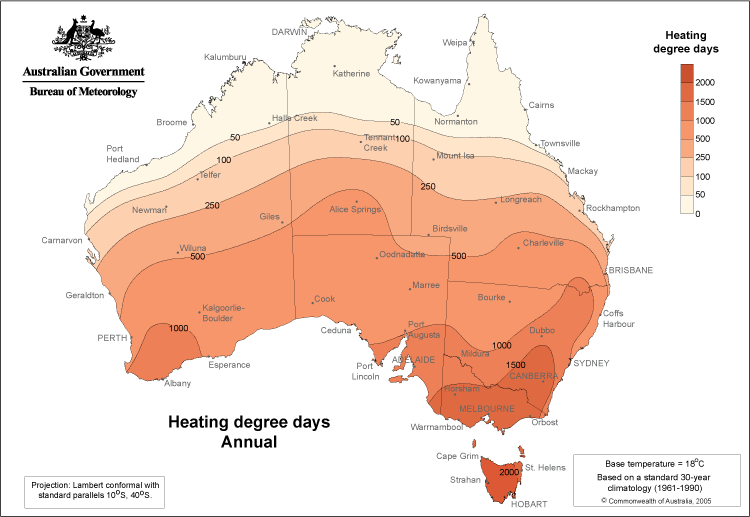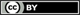# Average annual and monthly heating and cooling degree days

These heating and cooling degree days maps show the average annual or monthly number of days where temperature falls above or below a level of comfort.Product code: IDCJCM0017

## What do the maps show?

These maps show the average annual and average monthly number of heating and cooling degree days across Australia, over the period 1961 to 1990.

The number of cooling degree days increases towards the north of the continent, while the number of heating degree days increases towards the south of the continent, following the pattern of the mean temperature.

These maps are commonly used in the energy industry, as well as by air conditioning and heating manufacturers. For example, the average annual number of cooling degree days with a base temperature of 18 degrees for Melbourne is about 250, whereas in Brisbane it is approximately 1000. Provided a home has a similar structure in both cities, on average four times the energy would be required to cool a home in Brisbane than Melbourne.

## How are the values calculated?

Heating and cooling degree days are based on the average daily temperature. The average daily temperature is calculated as follows: [maximum daily temperature + minimum daily temperature] / 2.
If the average daily temperature falls below comfort levels, heating is required; and if it is above comfort levels, cooling is required.

The heating degree days or cooling degree days are determined by the difference between the average daily temperature and the comfort level temperature. The comfort level values used are 12 and 18 degrees Celsius for heating and 18 and 24 degrees Celsius for cooling.

For example, if heating is being considered to a temperature comfort level of 18 degrees, and the average daily temperature for a particular location was 14 degrees, then heating equivalent to 4 degrees (4 heating degree days) would be required to maintain a temperature of 18 degrees for that day. However if the average daily temperature was 20 degrees then no heating would be required, so the number of heating degree days for that day would be zero.

If cooling is being considered to a temperature comfort level of 24 degrees, and if the average temperature for a day was 27 degrees, then cooling equivalent to 3 degrees (3 cooling degree days) would be required to maintain a temperature of 24 degrees for that day. However if the average temperature was 21 degrees, then no cooling would be required, so the number of cooling degree days for that day would be zero.

Average annual heating and cooling degree days are calculated by adding heating and cooling degree days totals over a specified period (1961 to 1990) and dividing by the number of years in that period (30 years in this case). Similarly, average monthly heating and cooling degree days are calculated by adding monthly totals and dividing by the number of years in the specified period.Unless otherwise noted, all maps, graphs and diagrams in this page are licensed under the Creative Commons Attribution 4.0 International Licence